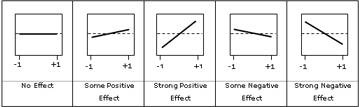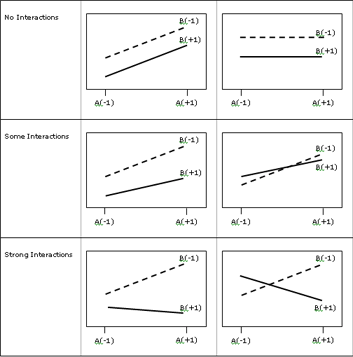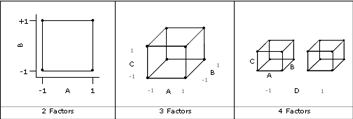# Factorial Plots

There are three types of factorial plots to help you visualize the effects, namely the Main Effects Plot, the Interactions Plot and the Cube Plot. They can be used to help you visualise how the response relates to one or more factors.

#### Main Effects Plot

The Main Effects Plot plots means of factor levels and it is used to visualise the magnitudes of main effects. The following figures show you how to interpret different types of Main Effects Plots.#### Interactions Plot

The Interactions Plot plots the means for each level of one factor with the levels of another factor. For more than two factors, a matrix of interaction plot is generated. It is used for identifying interactions between factors. The following figures show you how to interpret different types of Interactions Plot.#### Cube Plot

Cube plots are useful to visualise the limits (maximums and minimums) of the response for various combination of levels of factors. The following figure illustrates respective cube plots for 2, 3 and 4 factors. Each corner of the cube is the average response value for each combination of levels of factors. One can see that as the number of factors gets larger, cube plots may be too complex for useful application. It may be better to select 2 to 3 key factors to visualise in a cube plot at once.